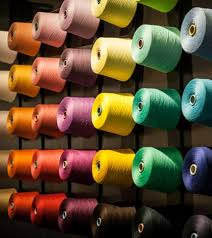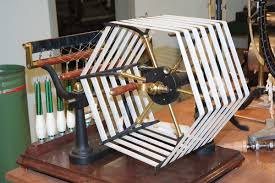Posted on

# Title of yarn

## Title of yarn

What is the title of yarn? It is the size of the dimension of the thread, that determines the thickness and consequently the quality of the fiber. Logically it shoud be the size of the diameter of the thread, but this is only possible for metal threads which are not deformable and have a constant section. So, how can we detrmine the diameter of a deformable and non constant section thread? Simple: we can use units that are easy to measure such as the length and weight. We can proceed in two ways: weight per lenght unit or viceversa; length per unit of weight; this type of measurement is called title.(Yarn)

Obviously, one of the two units has to be fixed, meanwhile the other one is variable. let’s get into the specifics; if it’s considered as a title, the weight relates to a fixed length, it is clear that the weight increases as the diameter of yarn increases; so the bigger the title the larger the thread diameter. (for example, 1000 yards of sewing machine thread is lighter than 1000 yards of rope). Viceversa, if we use as a title the length necessary to reach a certain weight, it’s obvious that the lenght increases when the thread diameter decreases. Consequently, the higher the title the thinner the thread ( to get one pound weight we need more sewing machine thread than rope.)

Consequently, the title of yarn is a number that indicates the thickness, in proportion to the thread section. There are two fondamental criteria for the measurement of yarn: direct and indirect. In the direct system, the title is proportional to the size of thread.(Machine to determine the length and the weight of the yarn)

## how to determine the title of yarn

### Direct title

1 Tex = 1 gram each km of yarn

1 Decitex (dtex) = 1 gram each 10 km of yarn (submultiple of Tex)

Denier (Td o den) = 1 gram each 9 km of Yarn

1 (Ts) = 1 pound each 14.400 Yard of yarn.

### Indirect title or number

Nm: indicates how many skein of yarn (1000 metres long) there are in 1 kg; it’s used for wool.

Nk: indicates how many metres of yarn there are in 1 kg.; it’s used for yarn and wool waste

Ne: indicates how many skein of yarn (840 yards) there are in 1 pound.

Nl:  indicates how many skein of yarn (300 yards) there are in 1 pound.

Nw: indicates how many skein of yarn (560 yards) there are in 1 pound.

Ns: indicates how many skein of yarn (256 yards) there are in 1 pound

Wikipedia